# Coherent waves can have different amplitudes

interference describes the superposition of two or more waves of any kind (i.e. sound, light, matter waves, etc.) according to the superposition principle (i.e. by adding the amplitudes, not the intensities). The waves cancel each other out, so one speaks of destructive interference. If the amplitudes increase, one speaks of constructive interference. This is shown using the example of sinusoidal waves of the same frequency with the same phase or phase shifted by 180 ° in the figure on the right. The pattern of points of constructive and destructive interference is called Interference pattern designated. A well-known example is the striped pattern behind a double-gap arrangement. The occurrence of interference in the physical experiment is considered to be evidence of the wave nature of the radiation being investigated. In a double slit experiment, for example, an interference pattern can also be observed when the light (photons) are replaced by electrons. Here one speaks of matter waves.

The word "interference" is just like the English "interference", from the old French "s'entreferir" (hit each other) derived. This old French word is derived from the Latin prefix "inter" (between) and the verb "ferire", (beat) composed.

### Beating and standing wave

If you superimpose two waves with unequal but close frequencies f1 and f2, the beating results in a pattern as shown in the first figure on the right (lower graph). A fast oscillation develops (frequency ffast = (f1 + f2)/2), which is amplitude modulated with a slow frequency (frequency fBeat = (f1 - f2)/2).

To tune musical instruments, you can change the corresponding setting until you no longer perceive a beat together with a reference tone (e.g. from a tuning fork). The measurement of beat signals can also be used to measure frequencies that are otherwise too high (for the measuring device). However, this requires a signal source that supplies signals with a very stable and precise frequency.

The interference of two waves of the same frequency but with opposite directions of propagation leads to a standing wave, also known as a standing wave.

### coherence

The wave field that arises from the interference of two (or more) arbitrary waves can only be stable over time if these waves have a (temporally) fixed phase relationship with one another. This is e.g. B. given when the waves come from the same source. One then speaks of coherent waves. If the waves are not monochromatic, i.e. consist of a whole series of frequency components, a coherence time is defined, which describes how the waves can be maximally shifted against each other in order to still generate a stable interference pattern. This coherence time (or the coherence length derived from it) is an important measure for physical light sources.

### Double slit test

A well-known experiment that illustrates the effect of interference is the double slit experiment (Thomas Young, 1802). A diaphragm consisting of two narrow (the order of magnitude of a wavelength) columns is set up in an electron or light beam. Behind it is a detector or a screen on which the electrons or photons are detected. If a gap is covered, a light stripe appears on the screen when illuminated, as expected. If both gaps are released, not only two strips of light appear, but several. The strip spacing is inversely proportional to the spacing of the column. Interference and diffraction lead to the fact that the waves of light are superimposed as described above and thus form an arrangement of light and dark stripes. The double-slit experiment is discussed again below in the section on interference in quantum mechanics.

### Interferometer

Interferometers are used in measurement technology. These use interference phenomena to measure lengths or phase shifts with a very high resolution. To do this, a (light) beam is split into two coherent parts, which are later superimposed again. The two rays lay different routes s1 and s2 back. If these differ by an integral multiple of the wavelength, constructive interference is obtained at the output of the interferometer. If they differ by half a wavelength (phase shift of 180 °), one obtains destructive interference. If you now set the interferometer first to constructive interference and then introduce an additional phase shift Δφ in one of the two arms, this can be determined via the intensity at the output of the interferometer.

There are different implementations of this principle: Mach-Zehnder interferometer, Michelson interferometer, Sagnac interferometer, etc.

### Diffraction and resolving power of optical devices

When waves are diffracted at a slit, a wavefront hits a slit. According to Huygens' principle, new spherical waves emanate from all points along the gap and interfere behind them. This creates the typical diffraction pattern of a single slit (see figure on the right). The wider the slit and the smaller the wavelength, the narrower the diffraction pattern.

Every optical device contains an entry opening (e.g. opening or first lens of an objective or main mirror of a telescope). This can be modeled as a slit and its diffraction pattern limits the resolution of the device. This limitation is based on the fact that every optical device generates an image of this entrance slit in some way, which is given by the width of the diffraction pattern.

### Interference colors

Interference colors can be observed on thin layers of optically transparent materials. They are created by superimposing the rays that are reflected on the surface of the layer and on the lower boundary surface.

In the picture on the right is ray 2 thrown back on the surface, ray 1 only after passing through the thin layer shown in blue. He kills you Δ = X0Y longer way back. Is Δ an odd multiple of half the wavelength λ, d. H.

Δ = (2n + 1) λ/ 2 for n = 0, 1, 2, ...

the rays are extinguished 1 and 2 out. The same laws apply as for interference at the double slit, just the other way around, since when ray 1 is reflected on the generally optically denser medium, a phase shift of half a wavelength occurs in the reflected ray. Beam 2 is reflected at the lower interface on the optically thinner medium, so that no phase jump occurs here. In the picture example it is blue light that interferes destructively. The light of other wavelengths is retained. If white light is radiated, it is reflected without any blue component. You can see yellow light, the complementary color to blue.

Examples of the occurrence of interference colors are given in the article Newton's rings.

### White light interference

The superposition of continuously varying wavelength and amplitude (spectrum) creates an interference pattern only within the coherence length. In white light interferometry, this behavior is used to obtain a clear length measurement. Another application example can be found in optical coherence tomography, which can thus capture three-dimensional structures.

### Laser speckle

Lasers are light sources with very high coherence (long coherence length, typically a few meters, up to a few hundred meters). This means that laser light is still capable of interference even after being reflected on diffuse surfaces. This explains the stable point pattern that is produced when a laser beam is reflected e.g. B. observed on a sheet of paper. The prerequisite for the appearance of speckle patterns is that the surface has a roughness in the order of magnitude of the wavelength of the laser light (with visible light: a few 100 nm).

### Anti-noise

In acoustics, interference is used to reduce disturbing noises, see anti-noise. This principle comes z. B. used in headphones for aircraft pilots to locally dampen machine noise.

### Mathematical representation

A wave is usually determined by a function of location x and time t written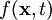. This expresses that a wave spreads both in space and in time. Several waves are now superimposedin one place x0, the wave field can be represented there as a superposition (sum) of the individual waves: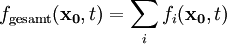### Interference between two waves of the same frequency and amplitude but different phase

The superposition of two waves with the same frequency and amplitude can be calculated using the trigonometric addition theorems. Become the two waves f1(t) and f2(t) with the common frequency ω, the amplitude a and the phases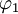and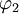by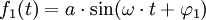and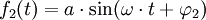described, then results for the resulting superposition of the waves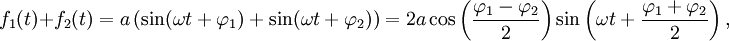d. H. a wave of the same frequency arises, the amplitude of which depends on the difference in the phases of the two original waves and whose phase is the mean of the phases of the original waves. For equal phases of the waves (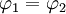) becomes the cosine one. The result is an amplitude of 2a, d. H. the amplitude doubles compared to the output amplitudes, which corresponds to constructive interference. For a phase difference of 180 °, (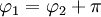) the cosine becomes zero, i.e. H. the resulting wave disappears. This corresponds to destructive interference.

### Interference between two waves of the same frequency but different amplitude and phase

For the same frequency of the waves, but different amplitudes and phases, the resulting wave can be calculated using pointer arithmetic. The two waves G1(t) and G2(t) have the common frequency ω, the amplitudes a1 and a2 and the phasesand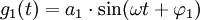and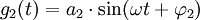The resulting superposition of the waves has the form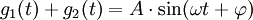with the amplitude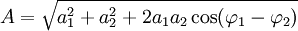and the phase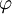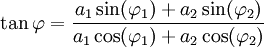.

### Superposition of circular waves

The figure on the left shows the interference of two circular wave groups of the same wavelength and amplitude. The crosses mark the position of the sources, the circles the maxima of the respective partial wave. Constructive interference occurs in white areas in a positive direction, in black constructive interference in a negative direction. There is destructive interference in the gray areas. It can be seen that the minima lie on a cluster of hyperbolas whose focal points are identical to the source locations of the waves. This is why we speak of hyperbolic interference in the case of two point sources. The hyperbola is the curve of all points that represent the transit time difference to the two source locations t = λ/ 2 have. The vertex distance 2a corresponds to the transit time difference 2a = (T1T2)v, if T1 and T2 represent the time reference of the two feeding time functions and v represents the medial speed of propagation.

### Clear explanation

In quantum mechanics, interference phenomena play a decisive role. Here particles (and more generally any states of a system) are described by wave functions. These are the solutions to the Schrödinger equation, which can take a form similar to a wave equation. Particles, i.e. matter, can thus behave like waves in quantum mechanics and also interfere (see also wave-particle dualism, matter waves). A well-known example is the interference of electrons in a double-slit experiment (see also the pictures on the right) or the interference of two Bose-Einstein condensates. Anton Zeilinger's group succeeded in observing an interference pattern of fullerenes (ball-shaped molecules made up of 60 carbon atoms). These are the heaviest particles for which an interference phenomenon has ever been observed.

### Mathematical version

In the Bra-Ket notation, any quantum mechanical state can be expressed in an orthonormal basis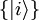(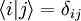) represent. There are those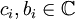complex coefficients: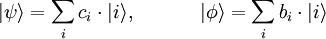For the likelihood of a system in the state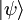in condition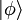what is measured is then: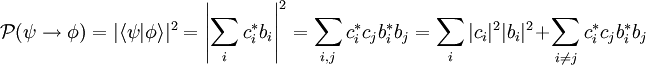It is important here that not the probabilities of the particle's location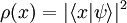are superimposed, but the (complex) wave functions themselves. If the probabilities of location were superimposed, one would lose the rear interference component in the above formula and the interference pattern would disappear.

De Broglie already postulated at the beginning of the century that all massive particles have a wavelength λ = H / p can be attributed to, wherein p the momentum of the particle is and H Planck's quantum of action. With this wavelength one can directly read the wave function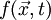for a particle and thus calculate the interference pattern using the methods described above for light.

1. Concise Oxford Dictionary, ISBN 0-198-61200-1
2. Katja Bammel: Sound against sound - active noise suppression, Physik Journal February 2007, pp.42-43
3. A Tonomura, J Endo, T Matsuda, T Kawasaki and H Ezawa: Demonstration of single-electron build-up of an interference pattern, American Journal of Physics 57: 117-120 (1989).
4. Markus Arndt, Olaf Nairz, Julian Voss-Andreae, Claudia Keller, Gerbrand van der Zouw and Anton Zeilinger: Wave particle duality of C60 molecules, Nature 401 (1999), 680-682, 

### literature

• Cohen-Tannoudji, Claude / Diu, Bernard / Laloë, Franck (1999):Quantum Mechanics 1 & 2, 2nd edition, Berlin - New York: Walter de Gruyter, ISBN 3-110-16458-2

Category: quantum physics